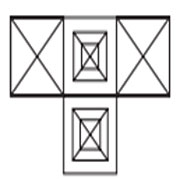## Construction Of Squares And Triangles

#### Construction Of Squares And Triangles

1. How many quadrilaterals are there in these given figures?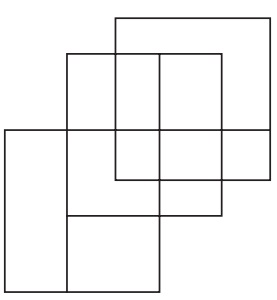1. TOTAL NUMBER OF QUADRILATERALS = 17

##### Correct Option: C

TOTAL NUMBER OF QUADRILATERALS = 17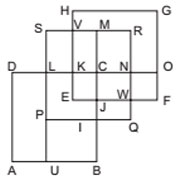1. Count the total number of Triangles of the following figures?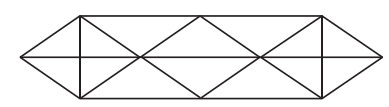1. The way to count the triangles of the figure is to draw the figure and count.

##### Correct Option: C

There are total 24 triangles. The way to count the triangles of the figure is to draw the figure and count.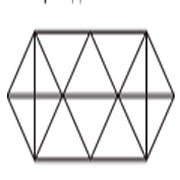1. Count the total number of triangles of the following figures?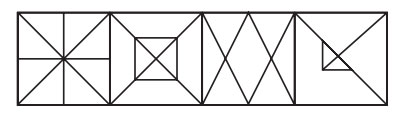1. See the figure carefully and count the number.

##### Correct Option: C

As per given figure, Please check the below number and count the triangles .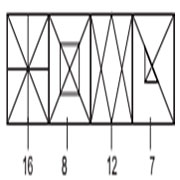1. How many squares and triangles are there in the given figures?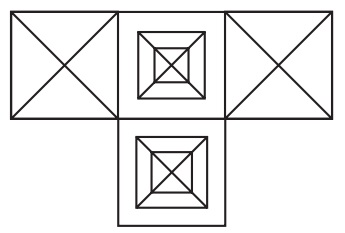1. The above figure contains 4 boxes were two are similar. This particular box contains 8 triangles and 1 square, as there are two boxes which are same, than there are total 16 triangles and 2 squares.

##### Correct Option: B

The above figure contains 4 boxes were two are similar. This particular box contains 8 triangles and 1 square, as there are two boxes which are same, then there are total 16 triangles and 2 squares.
The above figure contains :: (16 triangles and 3 squares) x 2 = 32 triangles and 6 squares.
Total triangles = 48 and total squares = 8